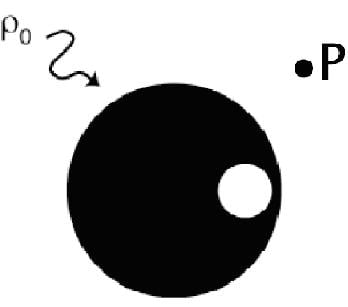# Electric field inside a cavity within a uniformly charged sphere

• semc
Thanks for catching that. :)In summary, the electric field within a cavity is uniform and due to symmetry, points in the direction of the center-to-center separation.

#### semc

A uniformly charged sphere (center 0,0) with radius 2a and volume charge density $$\sigma$$ has a cavity within it (center 0,a) with radius a. Show that the electric field within the cavity is uniform and Ex=0 Ey=$$\frac{a\sigma}{3\epsilon}$$ where \epsilon is the permittivity of free space.

Hi, I am able to prove the E field however I don't understand why we are only concern of the x and y-axis what about the z-axis? Why is E=Ey? Why is the E field of the cavity solely due to the y-axis? If that is the case won't the answer be the same if the question ask for a circle instead of a sphere?

•dumbdumNotSmart
There's no charge in the cavity? I must be misreading because this makes no sense to me. If there is no charge in the cavity, the electric field should be zero, shouldn't it?

Even if there is charge in the cavity, the electric field wouldn't be uniform, or at least it wouldn't be solely in the y-direction because of symmetry...

No charge in the cavity? I didn't say that. I only said the E-field in the x-direction is zero. Yes that is what i am trying to ask why the question says the E-field is only in the y-direction

•dumbdumNotSmart
Generally, a cavity is empty, so the natural assumption is that there is no charge in that region.

You must be leaving out or misstating some important information because with the problem as currently stated, the electric field should reflect the spherical symmetry in the problem.

vela said:
Generally, a cavity is empty, so the natural assumption is that there is no charge in that region.

You must be leaving out or misstating some important information because with the problem as currently stated, the electric field should reflect the spherical symmetry in the problem.

You're misunderstanding the problem. :) The cavity is shaped something like this:With the axes aligned a bit differently, and different numerical values for the radius of the cavity and the center-to-center distance of the cavity and the charged sphere.

The situation only has axial symmetry with respect to rotation about the y axis. There is no spherical symmetry to speak of.

I really liked this question when I first solved it in a class about gravitation. You get the same result there (Due to the analogous nature of the 1/r² gravitational field)
Interestingly enough, you get a similar result when you consider an infinitely long cylindrical current-carrying wire, with a cylindrical hole whose center is offset from the center of the wire.

There too you get a uniform (Both in magnitude and direction) $$\vec B$$ field inside the cavity. :) Quite remarkable!

I don't know how you've solved the problem, but I suggest that you do the following:
Solve it again, but this time give the cavity a parametric radius, and give the center-to-center separation a parametric radius as well. Don't align the center-to-center vector with any of the axes either.

In other words, try and solve the problem in the most general case. I assume the approach you've taken is to treat the cavity as an area that has super-positioned positive and negative charge densities of equal magnitudes (Yielding 0 net charge).

If you take the vector sum of the 2 sources of E-Field (Filling in the cavity with positive charge and negative charge means you only need to deal with a whole positive sphere centered at the origin, and a negative sphere centered at the center-to-center separation distance) you find that it is constant both in magnitude and direction.

The E-Field doesn't just point in the y-direction, it points in the direction of the center-to-center separation!
One could even guess that due to the axial symmetry of the situation, that the E-Field would be symmetrical with respect to rotation about that axis.
A field constant in magnitude and direction, however, is an incredible result! :)

Last edited:
•dumbdumNotSmart
You're right. I overlooked the info about where the cavity was centered.

•dumbdumNotSmart